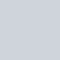# 前言

【Python 網頁爬蟲 #1】設定 google sheet API 並取得 json 金鑰，讓我們的資料能同步更新至雲端 google sheet 表格 (內含完整圖片說明)

# pygsheets 操作

pygsheets 已經幫我們把大部分的複雜功能簡化過了，因此我們可以很容易的使用 pygsheets 來操作 google sheets，

## 重要的前置步驟，共用 google sheet 編輯者 (讓程式可以編輯)## pygsheets 讀入 sheet

• 一個是我們剛剛下載下來的 json 金鑰，記得貼上對應的路徑位置
• 一個是你的 google sheet 網址 (看網址就知道了)，記得貼上
``````import pygsheets

auth_file = "xxxxxxxxxxxxxxxxxxxxxxxxxx.json"
gc = pygsheets.authorize(service_file = auth_file)

# setting sheet
sheet = gc.open_by_url(sheet_url)
``````

## pygsheets 從 sheet 找對應 工作表``````#選取by名稱
sheet_test01 = sheet.worksheet_by_title("test01")
``````

``````# read
A1 = sheet_test01.cell('A1')
print(A1)
print(A1.value)
``````
• 範例• 結果## pygsheets 表格寫入 (write)

``````# write
sheet_test01.update_value('A2', "test_A2") # 單一個
sheet_test01.update_values('B2', [['A', 'B', 'C', 'D']]) # 橫的
sheet_test01.update_values('A3', [['3'],['4'],['5'],['6']]) # 直的
``````
• 結果## pygsheets 表格插入 (insert)

(換句話說，我們如果填 1，「 1 不會動，會插入的是 2 」)

``````# insert
col = 1
sheet_test01.insert_cols(col, number=3) # add 2, 3, 4 (B, C, D)

row = 1
sheet_test01.insert_rows(row, number=3) # add 2, 3, 4
``````
• 結果### pygsheets 帶有值的表格插入 (insert with values)

(注意 values 必須是 matrix。)

``````# insert with values
values = [['M', 'N', 'O']] # matrix
insert_rows = 1 # add start from 2
sheet_test01.insert_rows(insert_rows, number=1, values=values)
``````
• 結果## pygsheets 表格結尾填入 (append)

``````# append
values = [['X', 'Y', 'Z']] # matrix
sheet_test01.append_table(values=values)
``````
• 結果# 最後，把自己常用的功能封裝，供以後方便使用

``````import pygsheets

auth_file = "xxxxxxxx.json"
gc = pygsheets.authorize(service_file = auth_file)
global_sheet = gc.open_by_url(sheet_url)

def __init__(self, sheet_name = "work01"):
# setting sheet
self.working_sheet = global_sheet.worksheet_by_title(sheet_name)

def write(self, position = "A1", content = "test"):
self.working_sheet.update_value(position, content)

content = self.working_sheet.cell(position)
print(content)
print(content.value)

def append(self, values = []):
self.working_sheet.append_table(values=values)

def insert(self, insert_rows = 1, values = []): # insert_rows = 1, start from 2
self.working_sheet.insert_rows(insert_rows, number=1, values=values)
``````

# Reference

⭐Python 基礎用法 相關文章整理⭐：
1.【Python】python list 清除, 移除內容元素 remove, pop, del, clear相關用法整理 sample code (內含範例程式碼)
2.【Python】寫模組 module、package 總整理 Importing files from different folder
3.【Python】python assert (斷言) 用法 sample code (內含範例程式碼)
4.【Python】python 一行 if else 語法 (one line if else) sample code (內含範例程式碼)
5.【Python】lambda 與 def function 使用方法與比較整理(內含範例程式碼)
6.【Python】python map 使用方法 與 其他寫法比較整理 (內含範例程式碼) sample code
7.【Python】python zip 使用方法 與 其他寫法比較整理 (內含範例程式碼) sample code
⭐Python 字串處理 相關文章整理⭐：
1.【Python】python print 變數的值 & 變數名稱 方法總整理
2.【Python】python string format str.format 總整理
⭐Python 檔案處理 相關文章整理⭐：
1.【Python】python 開關檔範例 與 程式模板 with open / file open sample code
2.【Python】取出檔案名稱 (含副檔名、不含副檔名) os path basename split 取出 檔名 路徑 不要副檔名 sample code
3.【Python】在 python 中利用 os.chmod 更改檔案的權限 chmod 777
4.【Python】利用 shutil 來複製檔案 shutil copy file
5.【Python】python 建立資料夾範例 mkdir os.makedirs() sample code
6.【Python】python 移除資料夾範例 rmdir shutil.rmtree() sample code
7.【Python】確認檔案是否存在 os.path.isfile / 確認資料夾是否存在 os.path.isdir sample code is folder / file exist
⭐Python 系統偵測 相關文章整理⭐：
1.【Python】python pyinotify sample code 偵測指定路徑底下的文件變化 (內有範例程式碼)
2.【Python】python 利用 argparse 使程式執行時可帶參數 (內附範例程式碼) sample code
⭐Python 平行運算 相關文章整理⭐：
1.【Python】threading – 建立多執行緒來執行程式 (內含範例程式碼) sample code
2.【Python】multiprocessing – 01 | 用多核心來執行程式 (內含範例程式碼) sample code
3.【Python】multiprocessing – 02 | pool, map, apply_async – 用多核心來執行程式並取得結果 (內含範例程式碼) sample code
5.【Python】python pooling multiprocess – 用多核心來執行程式 sample code (內含範例程式碼)
⭐Python 測試程式 相關文章整理⭐：
1.【Python】python 測試程式 – 利用 doctest 測試 python testcase 的優雅寫法，適用於 leetcode (doctest in function，搭配 function 的用法)
2.【Leetcode】python – 利用 doctest 測試 leetcode python testcase 的優雅寫法 (doctest in class，搭配 class 的用法)
⭐Python Chatbot 相關文章整理⭐：
1.【Chatbot】(全圖文說明) LINE Developers bot 機器人註冊與設定
2.【Chatbot】(全圖文說明) ngrok 本地伺服器設定方法 – LINE bot local server
3.【Chatbot】Dialogflow API 串接 python 的方法 (內含範例程式碼)
4.【Chatbot】[講義分享] 手把手實作line機器人 (linebot API 運用)
1.【Colab】Python colab 上傳檔案的方法 (內含範例程式碼) upload files
⭐Python 其他筆記 相關文章整理⭐：
1.【Python】anaconda 更新 (upgrade) python 3.8 版本筆記
2.【Sublime】Sublime 將縮排 “tab” 改成 4格空白 的方法 (圖文說明) sublime indent 4 spaces
3.【Sublime】Python 縮排小技巧 (很爛但實用) 快速將 tab 改成「4格空白」的方法
⭐【喜歡我的文章嗎? 歡迎幫我按讚~ 讓基金會請創作者喝一杯咖啡!### 1 則留言

1. #### 【Python】設定 google sheet API 並取得 json 金鑰，讓我們的資料能同步更新至雲端 google sheet 表格 (內含完整圖片說明) - 嗡嗡的隨手筆記

[…] 【Python】透過 pygsheets 操作 google sheet API，讓我們的資料能同步更新至雲端 go… […]